# Problem of the Day March 24, 2020

Mar 24, 2020March 24, 2020

Place the following decimals in order from least to greatest: 1.02, 1.002,  102.022, 1.2, 12.02,

Convert the following decimals into a simplified fraction and a percent.

.04, .45. 1.25

Simplify the following exponential expressions:

(2x)3, (2x2)3, (2x2)(23x3)2

Algebra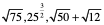Geometry

Name the 4 triangle congruence theorems and draw and example of each.

Algebra II

Convert the following quadratic equation into vertex form:

2x2 + 16x + 12

Trigonometry

Give the values for sin and cos of the following angles: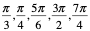PreCalculus

Find the vertical and horizontal asymptotes of the rational function without graphing: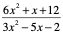Grade 6: 1.002, 1.02, 1.2, 12.02, 122.022

Grade 7: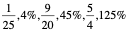Algebra: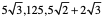Geometry: see this website https://www.appohigh.org/ourpages/auto/2014/11/17/63624959/Congruent%20Triangles.pdf

Algebra II:  2(x + 4)2 -20  vertex (-4, -20)

Trigonometry: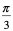sin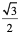cos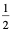,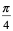sin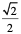cos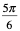sincos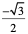,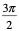sin  -1   cos  0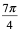sin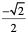cosPrecalculus: Vertical Asymptotes x =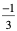and x = 2

Horizontal Asymptote y = 2